# Introduction

The aim of `factorMerger` is to provide a set of tools to support results from post hoc comparisons. Post hoc testing is an analysis performed after running ANOVA to examine differences between group means (of some response numeric variable) for each pair of groups (groups are defined by a factor variable).

This project arose from the need to create a method of post hoc testing which gives the hierarchical interpretation of relations between groups means. Thereby, for a given significance level we may divide groups into non-overlapping clusters.

# Algorithm inputs

In the current version the factorMerger package supports parametric models:

• one-dimensional Gaussian (with the argument `family = "gaussian"`),
• multi dimensional Gaussian (with the argument `family = "gaussian"`),
• binomial (with the argument `family = "binomial"`),
• survival (with the argument `family = "survival"`).

There are four algorithms available: adaptive, fast-adaptive, fixed and fast-fixed (they are set with the `method` argument of `mergeFactors`). Fast algorithms enable to unite only those groups whose group statistics (i.e. means in the Gaussian case) are close.

# Generating samples

To visualize functionalities of `factorMerger` we use samples or real data examples with response variable whose distribution follow one of the listed above. The corresponding factor variable is sampled uniformly from a finite set of a size \(k\).

To do so, we may use function `generateSample` or `generateMultivariateSample`.

``````library(factorMerger)
library(knitr)
library(dplyr)
randSample <- generateMultivariateSample(N = 100, k = 10, d = 3)``````

# Merging factors

`mergeFactors` is a function that performs hierarchical post hoc testing. As arguments it takes:

• matrix/data.frame/vector with numeric response,
• factor vector defining groups.

By default (with argument `abbreviate = TRUE`) factor levels are abbreviated and surrounded with brackets.

## Multi-dimensional Gaussian model

### Computations

``fmAll <- mergeFactors(response = randSample\$response, factor = randSample\$factor)``

`mergeFactors` outputs with information about the ‘merging history’.

``````mergingHistory(fmAll, showStats = TRUE) %>%
kable()``````
groupA groupB model pvalVsFull pvalVsPrevious
0 -411.9255 1.0000 1.0000
1 (I) (E) -411.9382 0.9991 0.9991
2 (A) (J) -412.0626 0.9997 0.9738
3 (I)(E) (A)(J) -412.3915 0.9997 0.8975
4 (D) (B) -412.9334 0.9996 0.8033
5 (G) (D)(B) -414.2715 0.9966 0.4797
6 (G)(D)(B) (F) -415.9719 0.9858 0.3647
7 (H) (I)(E)(A)(J) -418.1367 0.9529 0.2518
8 (G)(D)(B)(F) (C) -423.1086 0.6682 0.0234
9 (H)(I)(E)(A)(J) (G)(D)(B)(F)(C) -430.4130 0.1582 0.0028

Each row of the above frame describes one step of the merging algorithm. First two columns specify which groups were merged in the iteration, columns model and GIC gather loglikelihood and Generalized Information Criterion for the model after merging. Last two columns are p-values for the Likelihood Ratio Test – against the full model (pvalVsFull) and against the previous one (pvalVsPrevious).

If we choose a fast version of merging one dimensional response is fitted using `isoMDS{MASS}`. Next, in each step only groups whose means are closed are compared.

``````fm <- mergeFactors(response = randSample\$response, factor = randSample\$factor,
method = "fast-fixed")

mergingHistory(fm, showStats = TRUE) %>%
kable()``````
groupA groupB model pvalVsFull pvalVsPrevious
0 -411.9255 1.0000 1.0000
1 (I) (E) -411.9382 0.9991 0.9991
2 (I)(E) (A) -412.1038 0.9994 0.9607
3 (I)(E)(A) (J) -412.3915 0.9997 0.9143
4 (D) (B) -412.9334 0.9996 0.8033
5 (I)(E)(A)(J) (G) -415.2840 0.9776 0.2262
6 (I)(E)(A)(J)(G) (D)(B) -418.3399 0.8624 0.1264
7 (I)(E)(A)(J)(G)(D)(B) (F) -421.3636 0.6959 0.1264
8 (I)(E)(A)(J)(G)(D)(B)(F) (C) -426.9015 0.2673 0.0143
9 (H) (I)(E)(A)(J)(G)(D)(B)(F)(C) -430.4130 0.1582 0.0794

### Final clusters

Algorithms implemented in the factorMerger package enable to create unequivocal partition of a factor. Below we present how to extract the partition from the `mergeFactor` output.

• predict new labels for observations
``````cutTree(fm)
#>    (C)          (G)          (F)          (I)(E)(A)(J) (G)
#>    (I)(E)(A)(J) (I)(E)(A)(J) (D)(B)       (G)          (F)
#>   (D)(B)       (G)          (I)(E)(A)(J) (I)(E)(A)(J) (I)(E)(A)(J)
#>   (D)(B)       (I)(E)(A)(J) (D)(B)       (D)(B)       (C)
#>   (H)          (D)(B)       (D)(B)       (D)(B)       (D)(B)
#>   (F)          (H)          (F)          (I)(E)(A)(J) (G)
#>   (H)          (I)(E)(A)(J) (D)(B)       (I)(E)(A)(J) (G)
#>   (I)(E)(A)(J) (H)          (H)          (D)(B)       (I)(E)(A)(J)
#>   (I)(E)(A)(J) (I)(E)(A)(J) (I)(E)(A)(J) (I)(E)(A)(J) (C)
#>   (D)(B)       (G)          (H)          (I)(E)(A)(J) (C)
#>   (I)(E)(A)(J) (I)(E)(A)(J) (I)(E)(A)(J) (D)(B)       (I)(E)(A)(J)
#>   (I)(E)(A)(J) (G)          (I)(E)(A)(J) (F)          (D)(B)
#>   (I)(E)(A)(J) (F)          (D)(B)       (C)          (H)
#>   (C)          (H)          (G)          (I)(E)(A)(J) (G)
#>   (C)          (H)          (D)(B)       (F)          (I)(E)(A)(J)
#>   (I)(E)(A)(J) (F)          (I)(E)(A)(J) (D)(B)       (G)
#>   (D)(B)       (I)(E)(A)(J) (I)(E)(A)(J) (D)(B)       (I)(E)(A)(J)
#>   (D)(B)       (G)          (G)          (H)          (I)(E)(A)(J)
#>   (D)(B)       (I)(E)(A)(J) (H)          (F)          (I)(E)(A)(J)
#>   (G)          (C)          (I)(E)(A)(J) (D)(B)       (H)
#> Levels: (H) (I)(E)(A)(J) (G) (D)(B) (F) (C)``````

By default, `cutTree` returns a factor split for the optimal GIC (with penalty = 2) model. However, we can specify different metrics (`stat = c("loglikelihood", "p-value", "GIC"`) we would like to use in cutting. If `loglikelihood` or `p-value` is chosen an exact threshold must be given as a `value` parameter. Then `cutTree` returns factor for the smallest model whose statistic is higher than the threshold. If we choose `GIC` then `value` is interpreted as GIC penalty.

``````mH <- mergingHistory(fm, T)
thres <- mH\$model[nrow(mH) / 2]
cutTree(fm, stat = "loglikelihood", value = thres)
#>    (C)          (G)          (F)          (I)(E)(A)(J) (G)
#>    (I)(E)(A)(J) (I)(E)(A)(J) (D)(B)       (G)          (F)
#>   (D)(B)       (G)          (I)(E)(A)(J) (I)(E)(A)(J) (I)(E)(A)(J)
#>   (D)(B)       (I)(E)(A)(J) (D)(B)       (D)(B)       (C)
#>   (H)          (D)(B)       (D)(B)       (D)(B)       (D)(B)
#>   (F)          (H)          (F)          (I)(E)(A)(J) (G)
#>   (H)          (I)(E)(A)(J) (D)(B)       (I)(E)(A)(J) (G)
#>   (I)(E)(A)(J) (H)          (H)          (D)(B)       (I)(E)(A)(J)
#>   (I)(E)(A)(J) (I)(E)(A)(J) (I)(E)(A)(J) (I)(E)(A)(J) (C)
#>   (D)(B)       (G)          (H)          (I)(E)(A)(J) (C)
#>   (I)(E)(A)(J) (I)(E)(A)(J) (I)(E)(A)(J) (D)(B)       (I)(E)(A)(J)
#>   (I)(E)(A)(J) (G)          (I)(E)(A)(J) (F)          (D)(B)
#>   (I)(E)(A)(J) (F)          (D)(B)       (C)          (H)
#>   (C)          (H)          (G)          (I)(E)(A)(J) (G)
#>   (C)          (H)          (D)(B)       (F)          (I)(E)(A)(J)
#>   (I)(E)(A)(J) (F)          (I)(E)(A)(J) (D)(B)       (G)
#>   (D)(B)       (I)(E)(A)(J) (I)(E)(A)(J) (D)(B)       (I)(E)(A)(J)
#>   (D)(B)       (G)          (G)          (H)          (I)(E)(A)(J)
#>   (D)(B)       (I)(E)(A)(J) (H)          (F)          (I)(E)(A)(J)
#>   (G)          (C)          (I)(E)(A)(J) (D)(B)       (H)
#> Levels: (H) (I)(E)(A)(J) (G) (D)(B) (F) (C)``````

In this example data partition is created for the last model from the merging path whose loglikelihood is greater than -412.9334.

• get final clusters and clusters dictionary
``````getOptimalPartition(fm)
#>  "(H)"          "(I)(E)(A)(J)" "(G)"          "(D)(B)"
#>  "(F)"          "(C)"``````

Function `getOptimalPartition` returns a vector with the final cluster names from the factorMerger object.

``````getOptimalPartitionDf(fm)
#>    orig         pred
#> 1   (C)          (C)
#> 2   (G)          (G)
#> 3   (F)          (F)
#> 4   (E) (I)(E)(A)(J)
#> 6   (A) (I)(E)(A)(J)
#> 8   (D)       (D)(B)
#> 11  (B)       (D)(B)
#> 15  (J) (I)(E)(A)(J)
#> 21  (H)          (H)
#> 41  (I) (I)(E)(A)(J)``````

Function `getOptimalPartitionDf` returns a dictionary in a data frame format. Each row gives an original label of a factor level and its new (cluster) label.

Similarly to `cutTree`, functions `getOptimalPartition` and `getOptimalPartitionDf` take arguments `stat` and `threshold`.

### Visualizations

We may plot results using function `plot`.

``plot(fm, panel = "all", nodesSpacing = "equidistant", colorCluster = TRUE)``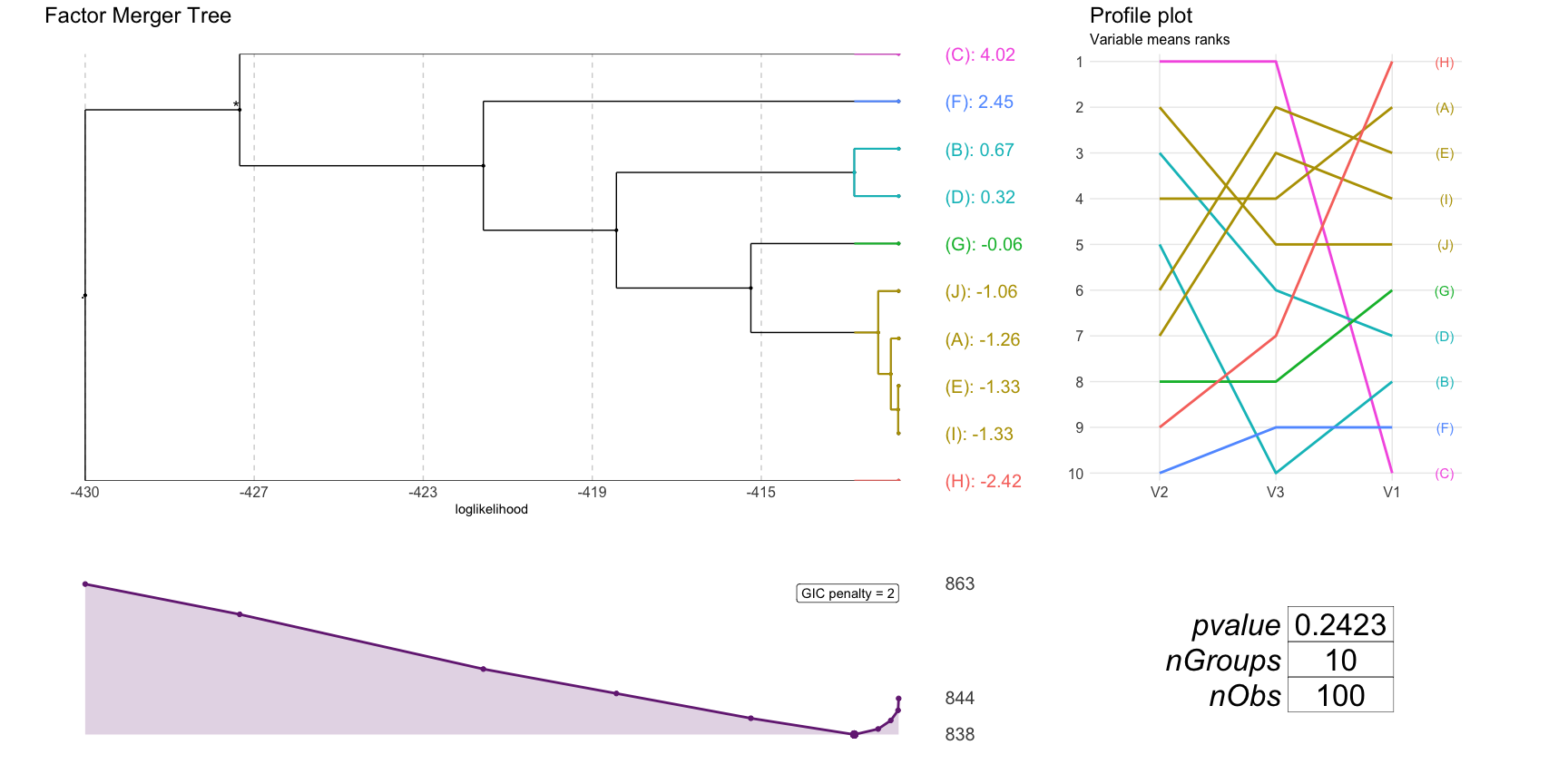``````plot(fmAll, panel = "tree", statistic = "p-value",
nodesSpacing = "effects", colorCluster = TRUE)``````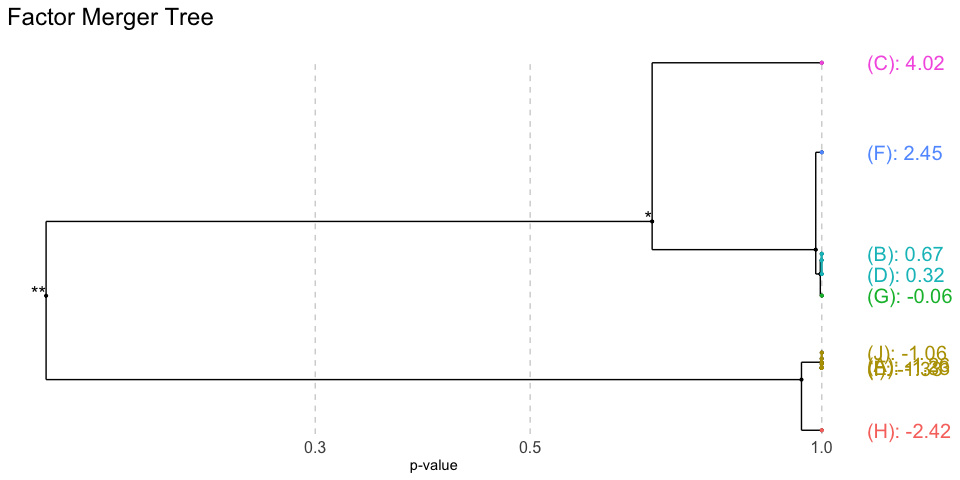``plot(fm, colorCluster = TRUE, panel = "response")``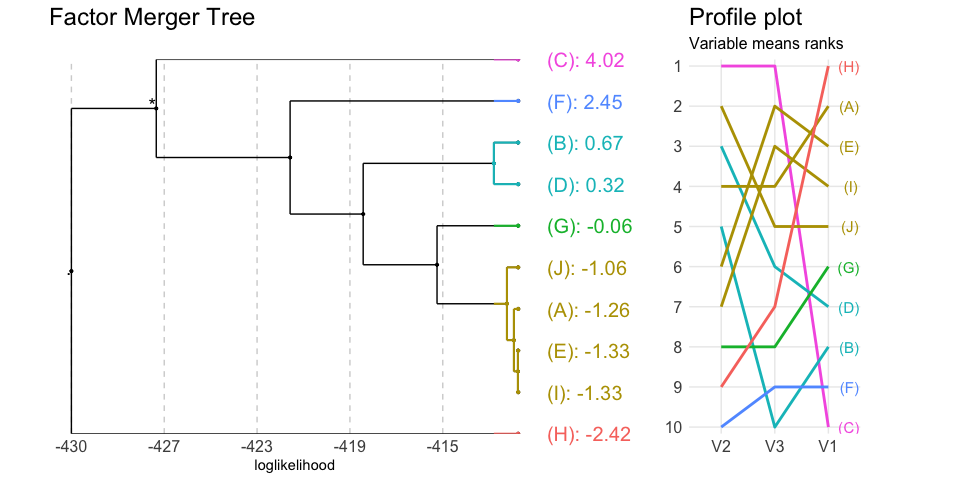The heatmap on the right shows means of all variables taken into analysis by groups.

``plot(fm, colorCluster = TRUE, panel = "response", responsePanel = "profile")``In the above plots colours are connected with the group. The plot on the right shows means rankings for all variables included in the algorithm.

It is also possible to plot GIC together with the merging path plot.

``plot(fm, panel = "GIC", penalty = 5)``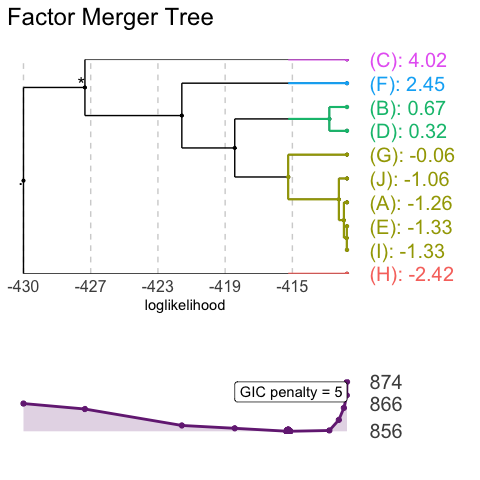Model with the lowest GIC is marked.

## One-dimensional Gaussian model

``oneDimRandSample <- generateSample(1000, 10)``
``````oneDimFm <- mergeFactors(response = oneDimRandSample\$response, factor = oneDimRandSample\$factor,
method = "fixed")
mergingHistory(oneDimFm, showStats = TRUE) %>%
kable()``````
groupA groupB model pvalVsFull pvalVsPrevious
0 -1395.130 1.0000 1.0000
1 (C) (H) -1395.130 0.9874 0.9874
2 (D) (B) -1395.131 0.9994 0.9752
3 (A) (J) -1395.133 0.9999 0.9442
4 (C)(H) (F) -1395.138 1.0000 0.9256
5 (C)(H)(F) (G) -1395.169 0.9999 0.8025
6 (D)(B) (C)(H)(F)(G) -1395.311 0.9992 0.5949
7 (A)(J) (I) -1395.799 0.9877 0.3243
8 (E) (D)(B)(C)(H)(F)(G) -1396.616 0.9374 0.2021
9 (E)(D)(B)(C)(H)(F)(G) (A)(J)(I) -1400.097 0.3612 0.0084
``plot(oneDimFm, palette = "Reds")``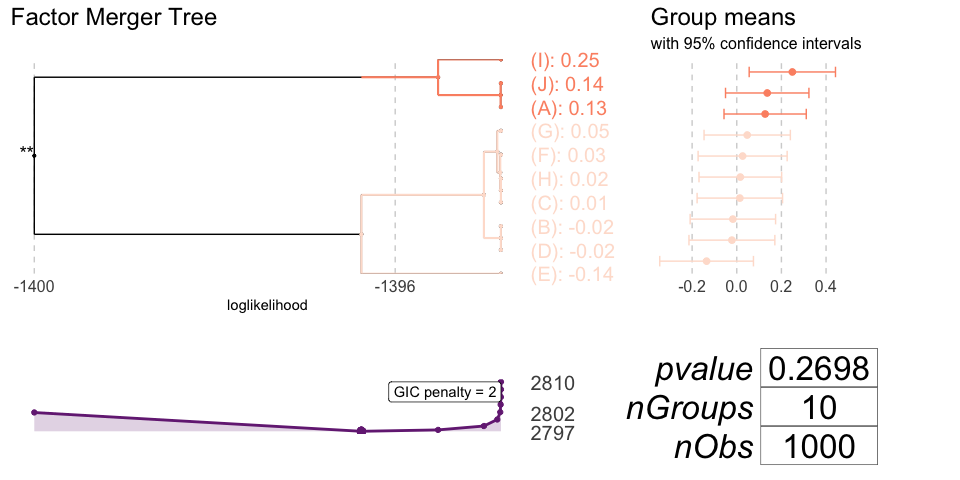``plot(oneDimFm, responsePanel = "boxplot", colorCluster = TRUE)``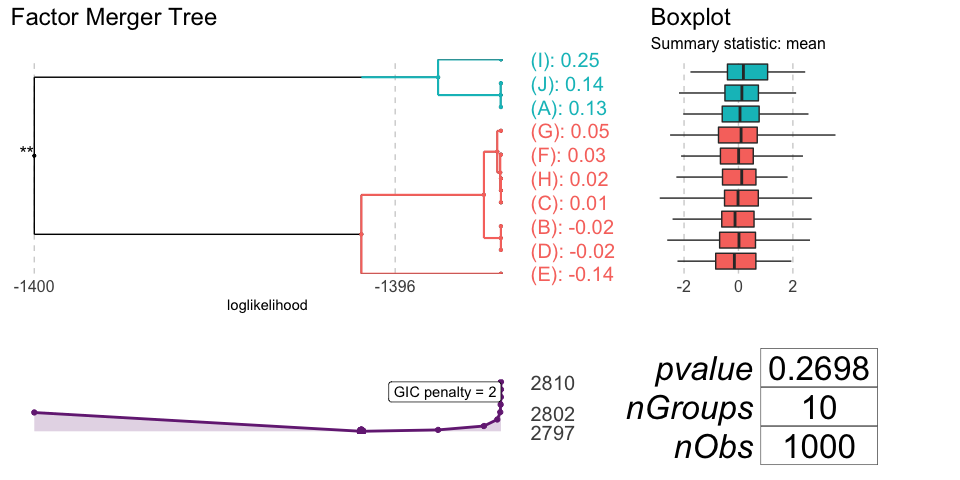## Binomial model

If `family = "binomial"` response must have to values: `0` and `1` (`1` is interpreted as success).

``````binomRandSample <- generateSample(1000, 10, distr = "binomial")
table(binomRandSample\$response, binomRandSample\$factor) %>%
kable()``````
J H G A D F C B I E
0 88 56 46 54 51 29 24 20 8 3
1 16 28 27 64 67 63 79 84 93 100
``````binomFm <- mergeFactors(response = binomRandSample\$response,
factor = binomRandSample\$factor,
family = "binomial",
mergingHistory(binomFm, showStats = TRUE) %>%
kable()``````
groupA groupB model pvalVsFull pvalVsPrevious
0 -513.9760 1.0000 1.0000
1 (A) (D) -514.0533 0.6943 0.6943
2 (H) (G) -514.1677 0.8256 0.6324
3 (C) (B) -514.4240 0.8264 0.4740
4 (I) (E) -515.7203 0.4796 0.1074
5 (F) (C)(B) -517.4859 0.2192 0.0602
6 (J) (H)(G) -523.9112 0.0029 0.0003
7 (A)(D) (F)(C)(B) -535.8790 0.0000 0.0000
8 (A)(D)(F)(C)(B) (I)(E) -572.9249 0.0000 0.0000
9 (J)(H)(G) (A)(D)(F)(C)(B)(I)(E) -663.5725 0.0000 0.0000
``plot(binomFm, colorCluster = TRUE, penalty = 7)``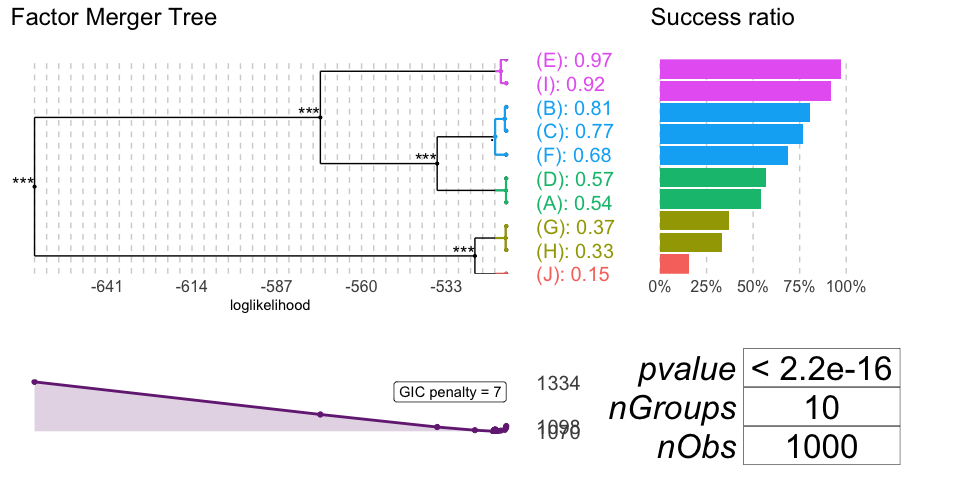``plot(binomFm, gicPanelColor = "red")``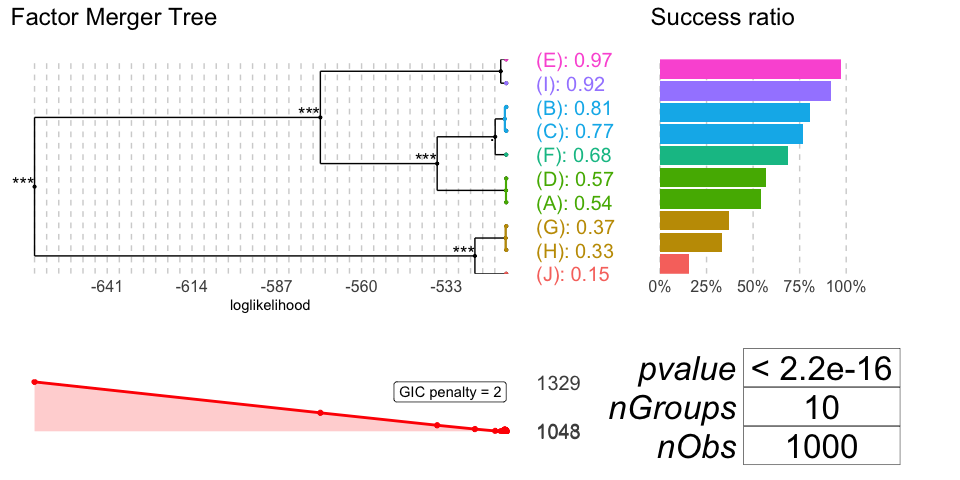## Survival model

If `family = "survival"` response must be of a class `Surv`.

`````` library(survival)
data(veteran)
survResponse <- Surv(time = veteran\$time,
event = veteran\$status)
survivalFm <- mergeFactors(response = survResponse,
factor = veteran\$celltype,
family = "survival")``````
`````` mergingHistory(survivalFm, showStats = TRUE) %>%
kable()``````
groupA groupB model pvalVsFull